# Examples in mathematics

Examples in mathematics have a different flavor than examples in other disciplines. This is probably because definitions in mathematics are different from definitions in other disciplines (mathematical definitions are exact). Some common problems of deciding whether something is or is not an example do not appear in mathematics. Instead, there are other problems.

## Unit testing and examples

A common problem in math is that one comes in with some preconceived idea of what an object should "look like" which is different from what the definition says. In other words, there is a mismatch between one's intuitive notion and the definition.

Take the example of a definition of function. A function is some object that takes each object in some set to a unique object in another set. Someone who was not familiar with the formal definition might mistakenly think of a function as "something that is defined by a formula".

In giving examples, it is particularly important to give examples in the places where intuition and the formal definition disagree. By default, the learner may have a tendency to search only for positive examples.

One can view the giving of examples as analogous to writing unit tests in programming. It is good to have some obvious examples, but one also wants to test the software on surprising cases (called "edge cases") to make sure the software really works.

There is a tendency in human thinking to leave ideas merely at the verbal level, i.e. at a level where the ideas don't constrain anticipation. Giving surprising examples and non-examples is one way to catch people's fuzzy thinking and to correct it.

(I think there's an analogy to overfitting that would be good to explain.)

Is an example according to definition Is not an example according to definition
Is an example according to intuition An "obvious" example, or central example. A surprising non-example. False positives, also known as type I errors.
Is not an example according to intuition A surprising example. False negatives, also known as type II errors. An obvious non-example.

### Obvious examples

An "obvious" example, or central example.

Let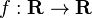$f : \mathbf R \to \mathbf R$ be defined by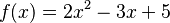$f(x) = 2x^2 - 3x + 5$. This does define a function, and someone who thought that a function is "something that is defined by a formula" would think that this is a function.

### Surprising non-examples

A surprising non-example. Let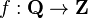$f : \mathbf Q \to \mathbf Z$ be defined by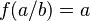$f(a/b) = a$ (i.e. a function that outputs the numerator of a fraction). This does not define a function. To see this, note that$f(1/2) = 1$ and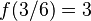$f(3/6) = 3$. But$1/2=3/6$ so we must have$f(1/2)=f(3/6)$ (a function must output a unique object for any given object), but$1\ne3$, so something has gone wrong. It turns out that each fraction has many different representations, and the idea of taking "the" numerator does not make sense, unless we constrain the representation somehow (e.g. by reducing the fraction and always putting any minus sign in the numerator). Someone who thought that a function is "something that is defined by a formula" might mistakenly think "this thing is defined by a formula, so must be a function".

As another example, let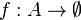$f : A \to \emptyset$ be a function where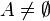$A \ne \emptyset$. This does not define a function. To see this, note that since$A\ne \emptyset$, we must have some$a \in A$. By the definition of function, we would have$f(a) \in \emptyset$, which is a contradiction since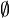$\emptyset$ is empty. Someone who was familiar with the empty function (see the next cell in this table) might conflate this example with it, and think that this is a function.

The examples in this cell are false positives, also known as type I errors.

### Surprising examples

A surprising example. Let$f : \mathbf N \to \mathbf N$ be defined by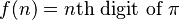$f(n) = n\text{th digit of }\pi$. This does define a function, but someone who thought that a function is "something that is defined by a formula" wouldn't think it is a function.

Another example is the empty function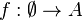$f : \emptyset \to A$ for any set$A$. This does define a function, but the function doesn't "do" anything. Since it is an "extreme" example of a function, someone who was only used to dealing with "normal-looking" functions (or someone who isn't used to working with the empty set or vacuous conditions) might dismiss this example.

As a third example, let$\mathcal M$ be the set of all Turing machines, and let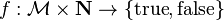$f : \mathcal M \times \mathbf N \to \{\text{true}, \text{false}\}$ be defined by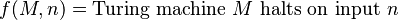$f(M,n) = \text{Turing machine }M\text{ halts on input }n$. This does define a function, although the function is not computable. Someone familiar with the halting problem might substitute "is a well-defined function" with "is a computable function" and say that this is not a function. In this example, it is not the intuitive notion of "function" that is getting in the way, but rather, a different technical concept (i.e., that of a computable function) that is getting in the way.

The examples in this cell are false negatives, also known as type II errors.

### Obvious non-examples

An obvious non-example. Let$f : \mathbf R \to \mathbf R$ be defined by$f(x) = x/0$. This does not define a function because division by zero is undefined. Someone familiar with division by zero would recognize this, and correctly reject this example.

## Hierarchical nature of examples

Something can be considered "concrete" or "abstract" depending on the context. Consider a term like "metric space". One can give examples of metric spaces. On the other hand, a metric space is itself an example (of a structured space, of a topological space).